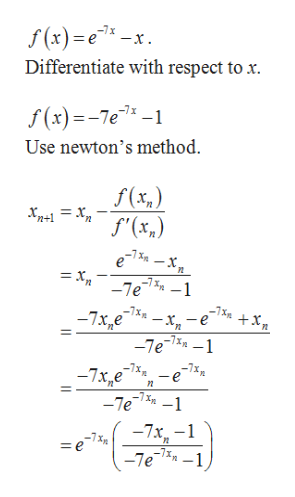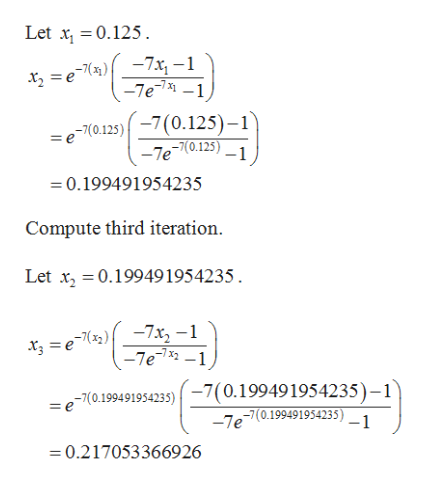# Use the Newton-Raphson method to find an approximate solution of the equatione-7x = xin the interval [0, 1]. Use the method until successive approximations obtained by a calculator are identical.x ≈

Question
3 views

Use the Newton-Raphson method to find an approximate solution of the equation

e-7x = x

in the interval [0, 1]. Use the method until successive approximations obtained by a calculator are identical.

x ≈
check_circle

Step 1

The functionhelp_outlineImage Transcriptionclosef(x)e-x Differentiate with respect to x -7x f(x)-7e-1 Use newton's method f(x,) -7x = X _]e1%n -1 -x,-e -7e1-1 -x 7x,e -7xm -7x,e -Te e -7x- -7e1-1 -7x = e fullscreen
Step 2

Compute first iteration.

Step 3

Compute second i...help_outlineImage TranscriptioncloseLet x 0.125 xz =e)-7x, -1 -7e-1 = e 7(0.125)7(0.125)-1 -Te(0.125)_1 0.199491954235 Compute third iteration Let x 0.199491954235 x, =e)-7x, -1 -7e-1 -7(0.1994 91954235)7(0.199491954235)-1 -Je7(0.199491954235)_1 = e =0.217053366926 fullscreen

### Want to see the full answer?

See Solution

#### Want to see this answer and more?

Solutions are written by subject experts who are available 24/7. Questions are typically answered within 1 hour.*

See Solution
*Response times may vary by subject and question.
Tagged in

### Other